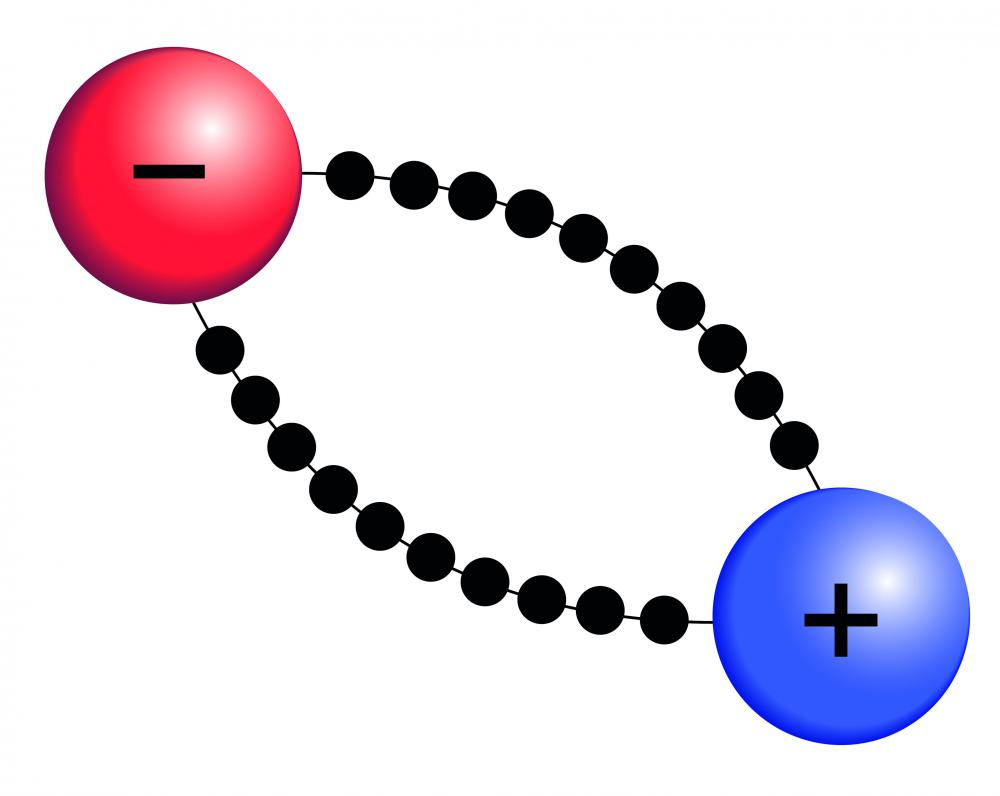# What Is an Electric Field?

Phil Riddel
Phil RiddelNegatively charged electrons are electrostatically bound to positively charged atomic nuclei.

An electric field can be regarded as the sphere of influence of an electrically charged object. Anything that has an electrical charge will affect, and be affected by, other charged bodies. If two charged objects are placed sufficiently close to one another, each will experience a measureable force acting upon it. The field is theoretically infinite in extent, but its magnitude diminishes with distance from the source according to the inverse square law. This means that if the distance is doubled, the strength of the field is divided by four, and at three times the distance, the strength is divided by nine, and so on; the field therefore becomes negligible at large distances.An electric field can be regarded as the sphere of influence of an electrically charged object.

Since an electric charge can be positive or negative, the electric field is a vector field, which means that it has a direction as well as a magnitude. Two electrically charged objects will experience a repulsive force if they have the same type of charge and an attractive force if they have different types of charge. The force experienced by a charged object in an electric field can be calculated as F = Eq, where F is the force in Newtons, E is the electric field in volts per meter (v/m) and q is the charge in Coulombs. This equation can be rearranged to give the strength of the field, E, in volts per meter: E = F/q. These examples apply to small, point-like, objects; for more complex, or multiple, charged bodies, the calculations are more complicated.

The direction of an electric field is defined as the direction in which the electric force would be felt by an object with a positive charge placed in the field. Thus, the field would point away from a positive charge and toward a negative charge, since like charges repel and unlike charges attract. In the case of two bodies with the same type of charge, each would experience a force — calculable by the F = Eq equation — directed away from the other object. Conversely, for two oppositely charged bodies, each would experience a force directed toward the other object.

An electric field line can be drawn with an arrow pointing away from a positive charge and pointing toward a negative charge. Thus, a positively charged object would be depicted with field lines pointing away from it in all directions, and a negatively charged object with field lines converging upon it. This, however, is just a convention and does not indicate that there is anything physical pointing in a particular direction.

The concept of an electric field as described above is part of “classical” physics. The classical description works well for everyday applications, but does not explain what is actually happening when charged objects attract or repel one another. A branch of quantum theory known as quantum electrodynamics (QED), attempts to do this in terms of the exchange of photons, the carriers of the electromagnetic force.

## You might also Like## Discuss this Article

Forgot password?
###### Register:
•Negatively charged electrons are electrostatically bound to positively charged atomic nuclei.
•An electric field can be regarded as the sphere of influence of an electrically charged object.